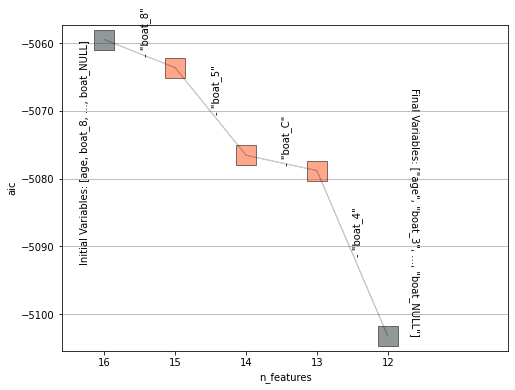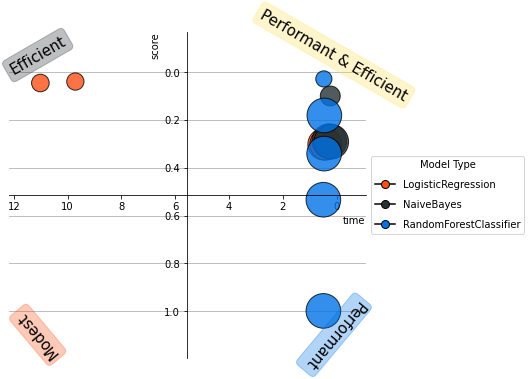# AutoML¶

In [ ]:
```class AutoML(name: str,
cursor=None,
estimator: (list, str) = "fast",
estimator_type: str = "auto",
metric: str = "auto",
cv: int = 3,
pos_label: (int, float, str) = None,
cutoff: float = -1,
nbins: int = 100,
lmax: int = 5,
optimized_grid: int = 2,
stepwise: bool = True,
stepwise_criterion: str = "aic",
stepwise_direction: str = "backward",
stepwise_max_steps: int = 100,
stepwise_x_order: str = "pearson",
preprocess_data: bool = True,
preprocess_dict: dict = {"identify_ts": False,},
print_info: bool = True,)
```

Tests multiple models to find the ones which maximize the input score.

### Parameters¶

Name Type Optional Description
name
str
Name of the model.
cursor
DBcursor
Vertica database cursor.
estimator
list / 'native' / 'all' / object
List of Vertica estimators with a fit method and a database cursor. Alternatively, you can specify 'native' for all native Vertica models, 'all' for all VerticaPy models and 'fast' for quick modeling.
estimator_type
str
Estimator Type.
• auto: Automatically detects the estimator type.
• regressor: The estimator will be used to perform a regression.
• binary: The estimator will be used to perform a binary classification.
• multi: The estimator will be used to perform a multiclass classification.
metric
str / list
Metric used to do the model evaluation.
• auto : logloss for classification & rmse for regression.

For Classification:
• accuracy : Accuracy
• auc : Area Under the Curve (ROC)
• bm : Informedness = tpr + tnr - 1
• csi : Critical Success Index = tp / (tp + fn + fp)
• f1 : F1 Score
• logloss : Log Loss
• mcc : Matthews Correlation Coefficient
• mk : Markedness = ppv + npv - 1
• npv : Negative Predictive Value = tn / (tn + fn)
• prc_auc : Area Under the Curve (PRC)
• precision : Precision = tp / (tp + fp)
• recall : Recall = tp / (tp + fn)
• specificity : Specificity = tn / (tn + fp)

For Regression:
• max : Max Error
• mae : Mean Absolute Error
• median : Median Absolute Error
• mse : Mean Squared Error
• msle : Mean Squared Log Error
• r2 : R-squared coefficient
• rmse : Root Mean Squared Error
• var : Explained Variance
cv
int
Number of folds.
pos_label
int / float / str
The main class to be considered as positive (classification only).
cutoff
float
The model cutoff (classification only).
nbins
int
Number of bins used to compute the different parameters categories.
lmax
int
Maximum length of each parameter list.
optimized_grid
int
If set to 0, the randomness is based on the input parameters. If set to 1, the randomness is limited to some parameters while others are picked based on a default grid. If set to 2, no randomness is used and a default grid is returned.
stepwise
bool
If True, the stepwise algorithm will be used to determine the final model list of parameters.
stepwise_criterion
str
Criterion used when doing the final estimator stepwise.
• aic: Akaike’s information criterion
• bic: Bayesian information criterion
stepwise_direction
str
Which direction to start the stepwise search. Can be done 'backward' or 'forward'.
stepwise_max_steps
int
The maximum number of steps to be considered when doing the final estimator stepwise.
x_order
str
Method to preprocess X before using the stepwise algorithm.
• pearson: X is ordered based on the Pearson's correlation coefficient.
• spearman: X is ordered based on Spearman's rank correlation coefficient.
• random: Shuffles the vector X before applying the stepwise algorithm.
• none: Does not change the order of X.
preprocess_data
bool
If True, the data will be preprocessed.
preprocess_dict
dict
Dictionary to pass to the AutoDataPrep class in order to preprocess the data before the clustering.
print_info
bool
If True, prints the model information at each step.

### Attributes¶

Name Type Description
preprocess_
object
Model used to preprocess the data.
best_model_
object
Most efficient models found during the search.
model_grid_
tablesample
Grid containing the different models information.

### Main Methods¶

Name Description
Trains the model.
Draws the AutoML Plot.

AutoML also inherits the vModel methods.

### Example¶

In :
```from verticapy.learn.delphi import AutoML

model = AutoML("titanic_autoML")
model.fit("public.titanic",
X = ["boat", "age", "fare", "pclass", "sex"],
y = "survived")
```
```Starting AutoML

```
```Testing Model - LogisticRegression

```
```Model: LogisticRegression; Parameters: {'tol': 1e-06, 'max_iter': 100, 'penalty': 'none', 'solver': 'bfgs'}; Test_score: 0.045092278134423; Train_score: 0.03266700850802573; Time: 11.01318351427714;
Model: LogisticRegression; Parameters: {'tol': 1e-06, 'max_iter': 100, 'penalty': 'l1', 'solver': 'cgd', 'C': 1.0}; Test_score: 0.301029995663981; Train_score: 0.301029995663981; Time: 0.4836126168568929;
Model: LogisticRegression; Parameters: {'tol': 1e-06, 'max_iter': 100, 'penalty': 'l2', 'solver': 'bfgs', 'C': 1.0}; Test_score: 0.0389093106215141; Train_score: 0.03761670311104077; Time: 9.713476419448853;
Model: LogisticRegression; Parameters: {'tol': 1e-06, 'max_iter': 100, 'penalty': 'enet', 'solver': 'cgd', 'C': 1.0, 'l1_ratio': 0.5}; Test_score: 0.301029995663981; Train_score: 0.301029995663981; Time: 0.40871278444925946;

Grid Search Selected Model
LogisticRegression; Parameters: {'solver': 'bfgs', 'penalty': 'l2', 'max_iter': 100, 'C': 1.0, 'tol': 1e-06}; Test_score: 0.0389093106215141; Train_score: 0.03761670311104077; Time: 9.713476419448853;

Testing Model - RandomForestClassifier

```
```Model: RandomForestClassifier; Parameters: {'max_features': 'max', 'max_leaf_nodes': 64, 'max_depth': 5, 'min_samples_leaf': 2, 'min_info_gain': 0.0, 'nbins': 32}; Test_score: 0.53332118474008; Train_score: 0.024024485689949998; Time: 0.49579938252766925;
Model: RandomForestClassifier; Parameters: {'max_features': 'auto', 'max_leaf_nodes': 1000, 'max_depth': 6, 'min_samples_leaf': 2, 'min_info_gain': 0.0, 'nbins': 32}; Test_score: 0.18075413063352067; Train_score: 0.03242237015931577; Time: 0.4595194657643636;
Model: RandomForestClassifier; Parameters: {'max_features': 'max', 'max_leaf_nodes': 128, 'max_depth': 5, 'min_samples_leaf': 1, 'min_info_gain': 0.0, 'nbins': 32}; Test_score: 0.998362104193236; Train_score: 0.018768792700085433; Time: 0.4953469435373942;
Model: RandomForestClassifier; Parameters: {'max_features': 'auto', 'max_leaf_nodes': 1000, 'max_depth': 5, 'min_samples_leaf': 2, 'min_info_gain': 0.0, 'nbins': 32}; Test_score: 0.34046297359628963; Train_score: 0.03411943678377016; Time: 0.4673316478729248;
Model: RandomForestClassifier; Parameters: {'max_features': 'max', 'max_leaf_nodes': 64, 'max_depth': 6, 'min_samples_leaf': 2, 'min_info_gain': 0.0, 'nbins': 32}; Test_score: 0.0280858921592775; Train_score: 0.028043079555751766; Time: 0.4781498908996582;

Grid Search Selected Model
RandomForestClassifier; Parameters: {'n_estimators': 10, 'max_features': 'max', 'max_leaf_nodes': 64, 'sample': 0.632, 'max_depth': 6, 'min_samples_leaf': 2, 'min_info_gain': 0.0, 'nbins': 32}; Test_score: 0.0280858921592775; Train_score: 0.028043079555751766; Time: 0.4781498908996582;

Testing Model - NaiveBayes

```
```Model: NaiveBayes; Parameters: {'alpha': 0.01}; Test_score: 0.29090422194048665; Train_score: 0.13004656759877523; Time: 0.20302971204121908;
Model: NaiveBayes; Parameters: {'alpha': 1.0}; Test_score: 0.28826599230322447; Train_score: 0.13364558821712672; Time: 0.27718393007914227;
Model: NaiveBayes; Parameters: {'alpha': 10.0}; Test_score: 0.0993037365388245; Train_score: 0.1357004188800761; Time: 0.23973441123962402;

Grid Search Selected Model
NaiveBayes; Parameters: {'alpha': 10.0, 'nbtype': 'auto'}; Test_score: 0.0993037365388245; Train_score: 0.1357004188800761; Time: 0.23973441123962402;

Final Model

RandomForestClassifier; Best_Parameters: {'n_estimators': 10, 'max_features': 'max', 'max_leaf_nodes': 64, 'sample': 0.632, 'max_depth': 6, 'min_samples_leaf': 2, 'min_info_gain': 0.0, 'nbins': 32}; Best_Test_score: 0.0280858921592775; Train_score: 0.028043079555751766; Time: 0.4781498908996582;

Starting Stepwise
```
```[Model 0] aic: -5059.450085323004; Variables: ['"age"', '"boat_8"', '"boat_5"', '"boat_3"', '"boat_14"', '"boat_10"', '"boat_C"', '"boat_4"', '"boat_15"', '"boat_13"', '"fare"', '"pclass"', '"boat_Others"', '"sex_male"', '"sex_female"', '"boat_NULL"']
[Model 1] aic: -5063.611150260146; (-) Variable: "boat_8"
[Model 2] aic: -5076.533381540704; (-) Variable: "boat_5"
[Model 3] aic: -5078.802513562311; (-) Variable: "boat_C"
[Model 4] aic: -5103.234091001247; (-) Variable: "boat_4"

Selected Model

[Model 4] aic: -5103.234091001247; Variables: ['"age"', '"boat_3"', '"boat_14"', '"boat_10"', '"boat_15"', '"boat_13"', '"fare"', '"pclass"', '"boat_Others"', '"sex_male"', '"sex_female"', '"boat_NULL"']
```
Out:model_type avg_score avg_train_score avg_time score_std score_train_std 1 RandomForestClassifier 0.0280858921592775 0.028043079555751766 0.4781498908996582 0.002281626426933572 0.002116431010659495 2 LogisticRegression 0.0389093106215141 0.03761670311104077 9.713476419448853 0.009640131017117106 0.005182382176420538 3 LogisticRegression 0.045092278134423 0.03266700850802573 11.01318351427714 0.012127985139832029 0.0017101929930854007 4 NaiveBayes 0.0993037365388245 0.1357004188800761 0.23973441123962402 0.029317107319519413 0.06609576307272419 5 RandomForestClassifier 0.18075413063352067 0.03242237015931577 0.4595194657643636 0.24997816858137195 0.0017359006195464433 6 NaiveBayes 0.28826599230322447 0.13364558821712672 0.27718393007914227 0.38688552822035643 0.12963854944028425 7 NaiveBayes 0.29090422194048665 0.13004656759877523 0.20302971204121908 0.40608893582798733 0.12025993490836732 8 LogisticRegression 0.301029995663981 0.301029995663981 0.4836126168568929 0.0 0.0 9 LogisticRegression 0.301029995663981 0.301029995663981 0.40871278444925946 0.0 0.0 10 RandomForestClassifier 0.34046297359628963 0.03411943678377016 0.4673316478729248 0.5169745181844193 0.005971065403523417 11 RandomForestClassifier 0.53332118474008 0.024024485689949998 0.49579938252766925 0.3254200624106811 0.0019006685797809384 12 RandomForestClassifier 0.998362104193236 0.018768792700085433 0.4953469435373942 0.7011511755098827 0.007677837096366947
Rows: 1-12 | Columns: 8
In :
```model.plot("stepwise")
```
Out:
`<AxesSubplot:xlabel='n_features', ylabel='aic'>`In :
```model.plot()
```
Out:
`<AxesSubplot:xlabel='time', ylabel='score'>`